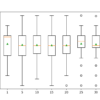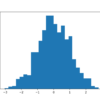# Archive | Data Preparation## How to Use StandardScaler and MinMaxScaler Transforms in Python

Many machine learning algorithms perform better when numerical input variables are scaled to a standard range. This includes algorithms that use a weighted sum of the input, like linear regression, and algorithms that use distance measures, like k-nearest neighbors. The two most popular techniques for scaling numerical data prior to modeling are normalization and standardization. […]## How to Perform Feature Selection for Regression Data

Feature selection is the process of identifying and selecting a subset of input variables that are most relevant to the target variable. Perhaps the simplest case of feature selection is the case where there are numerical input variables and a numerical target for regression predictive modeling. This is because the strength of the relationship between […]## How to Perform Feature Selection With Numerical Input Data

Feature selection is the process of identifying and selecting a subset of input features that are most relevant to the target variable. Feature selection is often straightforward when working with real-valued input and output data, such as using the Pearson’s correlation coefficient, but can be challenging when working with numerical input data and a categorical […]## Iterative Imputation for Missing Values in Machine Learning

Datasets may have missing values, and this can cause problems for many machine learning algorithms. As such, it is good practice to identify and replace missing values for each column in your input data prior to modeling your prediction task. This is called missing data imputation, or imputing for short. A sophisticated approach involves defining […]## Test-Time Augmentation For Tabular Data With Scikit-Learn

Test-time augmentation, or TTA for short, is a technique for improving the skill of predictive models. It is typically used to improve the predictive performance of deep learning models on image datasets where predictions are averaged across multiple augmented versions of each image in the test dataset. Although popular with image datasets and neural network […]## How to Use Polynomial Feature Transforms for Machine Learning

Often, the input features for a predictive modeling task interact in unexpected and often nonlinear ways. These interactions can be identified and modeled by a learning algorithm. Another approach is to engineer new features that expose these interactions and see if they improve model performance. Additionally, transforms like raising input variables to a power can […]## How to Scale Data With Outliers for Machine Learning

Many machine learning algorithms perform better when numerical input variables are scaled to a standard range. This includes algorithms that use a weighted sum of the input, like linear regression, and algorithms that use distance measures, like k-nearest neighbors. Standardizing is a popular scaling technique that subtracts the mean from values and divides by the […]## Recursive Feature Elimination (RFE) for Feature Selection in Python

Recursive Feature Elimination, or RFE for short, is a popular feature selection algorithm. RFE is popular because it is easy to configure and use and because it is effective at selecting those features (columns) in a training dataset that are more or most relevant in predicting the target variable. There are two important configuration options […]## How to Use Discretization Transforms for Machine Learning

Numerical input variables may have a highly skewed or non-standard distribution. This could be caused by outliers in the data, multi-modal distributions, highly exponential distributions, and more. Many machine learning algorithms prefer or perform better when numerical input variables have a standard probability distribution. The discretization transform provides an automatic way to change a numeric […]## How to Use Quantile Transforms for Machine Learning

Numerical input variables may have a highly skewed or non-standard distribution. This could be caused by outliers in the data, multi-modal distributions, highly exponential distributions, and more. Many machine learning algorithms prefer or perform better when numerical input variables and even output variables in the case of regression have a standard probability distribution, such as […]